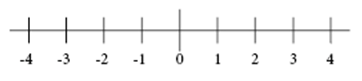# Integers and rational numbers

Natural numbers are all numbers 1, 2, 3, 4… They are the numbers you usually count and they will continue on into infinity.

Whole numbers are all natural numbers including 0 e.g. 0, 1, 2, 3, 4…

Integers include all whole numbers and their negative counterpart e.g. …-4, -3, -2, -1, 0,1, 2, 3, 4,…

All integers belong to the rational numbers. A rational number is a number

$\frac{a}{b},\: b\neq 0$

Where a and b are both integers.

Example

The number 4 is an integer as well as a rational number. As it can be written without a decimal component it belongs to the integers. It is a rational number because it can be written as:

$\frac{4}{1}$

or

$\frac{8}{2}$

or even

$\frac{-8}{-2}$

Whereas

$\frac{1}{5}=0.2$

is a rational number but not an integer.

A rational number written in a decimal form can either be terminating as in:

$\frac{1}{5}=0.2$

Or repeating as in

$\frac{5}{6}=0.83333...$

All rational numbers belong to the real numbers.

If you look at a numeral lineYou notice that all integers, as well as all rational numbers, are at a specific distance from 0. This distance between a number x and 0 is called a number's absolute value. It is shown with the symbol

$\left | x \right |$

If two numbers are at the same distance from 0 as in the case of 10 and -10 they are called opposites. Opposites have the same absolute value since they are both at the same distance from 0.

$\left | 10 \right |=10=\left | -10 \right |$

## Video lesson

Which of these rational numbers are integers?